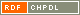# Show document

Title: On cryptographically significant mappings over GF(2 [sup] n) Pašalić, Enes (Author)http://dx.doi.org/10.1007/978-3-540-69499-1_16 English Not categorized 1.08 - Published Scientific Conference Contribution FAMNIT - Faculty of Mathematics, Science and Information Technologies In this paper we investigate the algebraic properties of important cryptographic primitives called substitution boxes (S-boxes). An S-box is a mapping that takes ▫$n$▫ binary inputs whose image is a binary ▫$m$▫-tuple; therefore it is represented as ▫$F:\text{GF}(2)^n \rightarrow \text{GF}(2)^m$▫. One of the most important cryptographic applications is the case ▫$n = m$▫, thus the S-box may be viewed as a function over ▫$\text{GF}(2^n)$▫. We show that certain classes of functions over ▫$\text{GF}(2^n)$▫ do not possess a cryptographic property known as APN (AlmostPerfect Nonlinear) permutations. On the other hand, when ▫$n$▫ is odd, an infinite class of APN permutations may be derived in a recursive manner, that is starting with a specific APN permutation on ▫$\text{GF}(2^k), k$▫ odd, APN permutations are derived over ▫$\text{GF}(2^{k+2i})$▫ for any ▫$i \geq 1$▫. Some theoretical results related to permutation polynomials and algebraic properties of the functions in the ring ▫$\text{GF}(q)[x,y]$▫ are also presented. For sparse polynomials over the field ▫$\text{GF}(2^n)$▫, an efficient algorithm for finding low degree I/O equations is proposed. cryptoanalysis, cryptography, permutation polynomials, power mappings, APN functions, S-box, CCZ-equivalence, algebraic properties 2008 Str. 189-204 512.624.95 151191931370 27Document is not linked to any category.

Average score: (0 votes) Voting is allowed only to logged in users.Hover the mouse pointer over a document title to show the abstract or click on the title to get all document metadata.

## Secondary language

Language: English kriptoanaliza, kriptografija, algebraične lastnosti